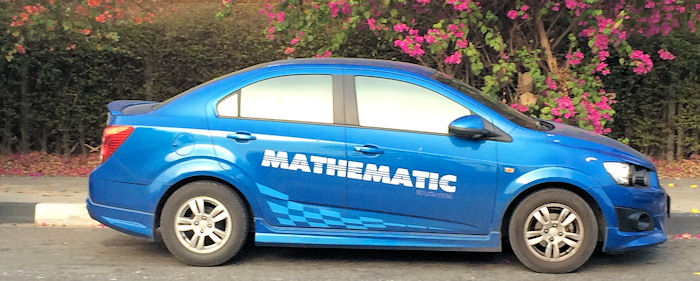# Exam-Style Questions on Percentages

## Problems on Percentages adapted from questions set in previous Mathematics exams.

### 1.

GCSE Higher

(a) In an election, David Linewhip gained 27 552 votes out of a total of 43 715 votes. Write 27 552 as a percentage of 43 715 giving your answer to the nearest integer.

(b) Goodtrip Travel increases the cost of a holiday by 8%. The holiday originally cost £1245. Find the new cost of the holiday.

### 2.

GCSE Higher

The value of a boat is £220 000.

Each year the value decreases by 12% of the value at the beginning of that year.

Work out the value of the boat after four years have passed.

### 3.

GCSE Higher

In 2009 the price of a Big Mac was £2.29.

In 2013 the price of a Big Mac had risen to £2.69.

(a) Work out the percentage increase in the price of this snack over the four years.

(b) Express the price in 2009 as a percentage of the 2013 price.

(c) By what percentage must the 2013 price be discounted to equal the 2009 price?

### 4.

IGCSE Extended

In January, a supermarket sold 12 624 boxes of breakfast cereal.

(a) The cost of a box of cereal was £2.15 . Calculate the amount received from the sale of the 12 624 boxes. Give your answer correct to the nearest hundred pounds.

(b) The number of boxes sold in February was 13% more than the number sold in January. Calculate the number of boxes sold in February.(c) There were 3 different types of cereal. The number of boxes sold of each type every month was in the ratio Riceflakes : Cornflakes : Branflakes = 4 : 5 : 3. The total number of boxes sold was 12 624. Calculate the number of boxes of Branflakes sold.

(d) How may more boxes of Cornflakes than Riceflakes were sold in February?

(e) $$\frac{5}{6}$$ of the 12 624 bottles sold in January are included in a special promotion. Calculate the number of boxes that are included in that promotion.

### 5.

GCSE Higher

Windthrup bought a car for £9500 which depreciated by 6% in the first year and 3.5% in the second year.

a) Windthrup thinks that in two years his car depreciated by 9.5% over the two years. Show, with any necessary calculations, that Winthrup is wrong.

b) Windthrup's car depreciates by $$x%$$ during the third year. At the end of the three years the car is worth £8190. Find the value of $$x$$ to three significant figures.### 6.

GCSE Higher

Zoe invests £5000 in an account for one year. At the end of the year, interest is added to her account.

Zoe pays tax on this interest at a rate of 25%. After the tax has been taken off she received £25.40 interest.

Work out the percentage interest rate for the account.

### 7.

GCSE Higher

John times how long it takes him to run around Hazelnut Park each Friday afternoon. The last three weeks his times, rounded to the nearest minute are $$p, q \; \text{and} \; r$$.

He notices that $$q$$ is 5% more than $$p$$ and $$r$$ is 5% less than $$q$$.

Indicate which of the following statements are true:

(a) $$r \lt p$$ and $$r \lt q$$

(b) $$r \gt p$$ and $$r \gt q$$

(c) $$r = p$$ and $$r \lt q$$

(d) $$p \gt q$$ and $$p = r$$

(e) $$p =q$$ and $$p \gt r$$

If you would like space on the right of the question to write out the solution try this Thinning Feature. It will collapse the text into the left half of your screen but large diagrams will remain unchanged.

The exam-style questions appearing on this site are based on those set in previous examinations (or sample assessment papers for future examinations) by the major examination boards. The wording, diagrams and figures used in these questions have been changed from the originals so that students can have fresh, relevant problem solving practice even if they have previously worked through the related exam paper.

The solutions to the questions on this website are only available to those who have a Transum Subscription.

Exam-Style Questions Main Page

Search for exam-style questions containing a particular word or phrase:

To search the entire Transum website use the search box in the grey area below.